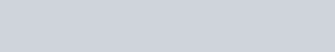A child has die whose 6 faces show the letters given below.
Question:

A child has die whose 6 faces show the letters given below.The die is thrown once. What is the probability of getting (i) A, (ii) B?

Solution:

When the die is thrown once, then any one of the six faces can show up.

∴ Total number of outcomes = 6

(i) There are 3 faces on the die showing the letter A.

$\therefore \mathrm{P}($ Getting the letter $\mathrm{A})=\frac{\text { Favourable number of outcomes }}{\text { Total number of outcomes }}=\frac{3}{6}=\frac{1}{2}$

(ii) There are 2 faces on the die showing the letter B.

Favourable number of outcomes = 2

$\therefore \mathrm{P}($ Getting the letter $\mathrm{B})=\frac{\text { Favourable number of outcomes }}{\text { Total number of outcomes }}=\frac{2}{6}=\frac{1}{3}$

Favourable number of outcomes = 3